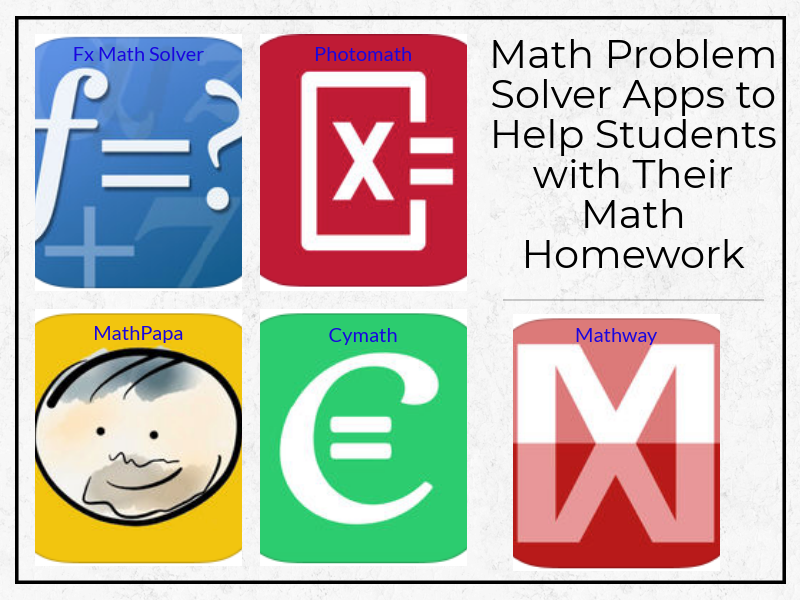# Math Problem Solver Apps to Help Students with Their Math Homework

Below is a collection of some good iPad apps to help students with their math homework. They provide detailed instructions on how to solve a wide variety of math problems covering different mathematical topics from algebra and trigonometry to complex calculus and statistics. The main educational strength of these apps is that by walking students through math solutions, students get to enhance their mathematical thinking, develop new skills and learn from the process. Some of these apps are featured here for the first time. We invite you to check them out and share with your students.1- Mathway
‘Mathway instantly solves your most difficult math problems - simply type your problem in (or point your camera and snap a pic!) to receive instant free answers. Need detailed step-by-step solutions? Mathway is like a private tutor in the palm of your hand, providing instant homework help anywhere, anytime.’

2- Photomath
‘Simply point your camera toward a math problem and Photomath will magically show the result with a detailed step-by-step instructions.’

3- Cymath
‘the Cymath app uses the same math engine while letting you solve problems on the go! Just enter your problem, and let Cymath solve it for you step-by-step. You can also use the camera to capture a problem without typing. We cover a wide variety of topics in algebra, calculus, and graphing.’

4- MathPapa